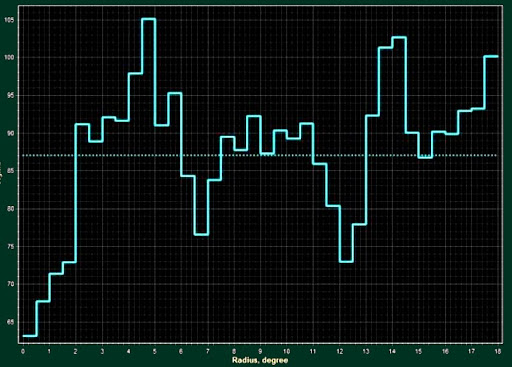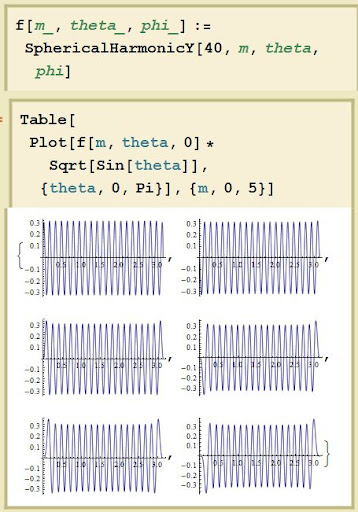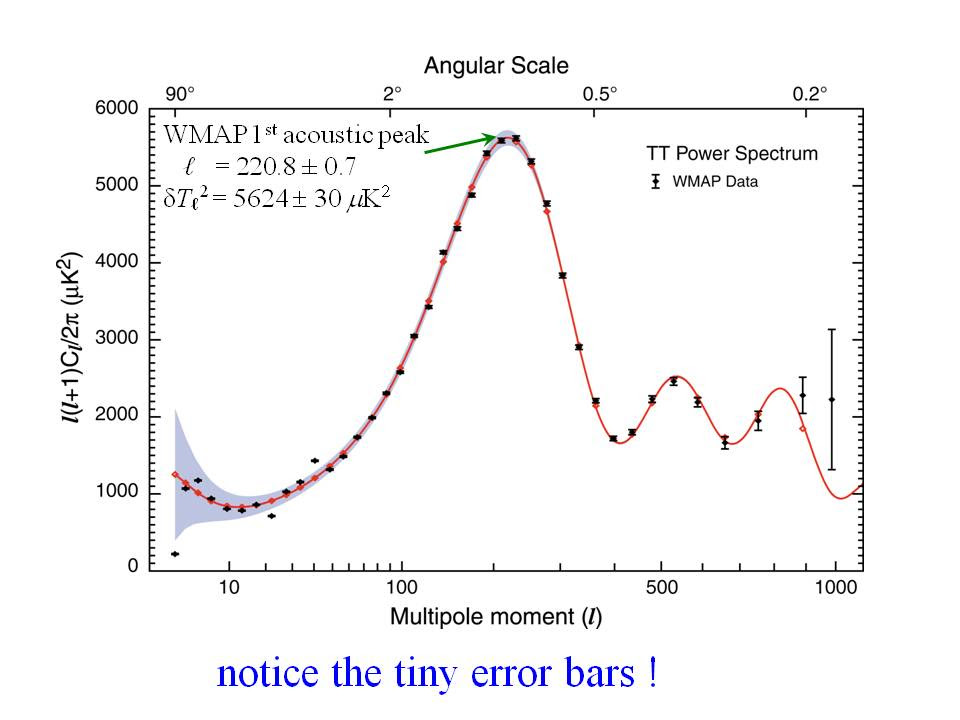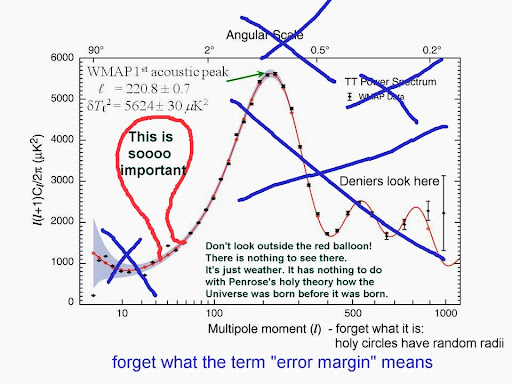## Monday, November 29, 2010 ...//### What Penrose and Gurzadyan have rediscovered is the WMAP excess at L=40

...the abundance of all other patterns ("random concentric circles") they may be observing statistically coincides with the standard WMAP prediction...I have been making more explicit and quantitative calculations of the claims by Penrose and Gurzadyan and I finally understood what they actually see.

The explanation why the effect is there remains mysterious but what the effect actually is, in the usual terminology of cosmologists, is totally clear to me now. First, let us ask what is the spacing of their concentric circles.Click to zoom in.

The graph above is borrowed from their paper. There are several similar graphs in the paper. You may see that the apparent radii of the concentric circles may be estimated as 4°, 9°, 14°, 19°. So the spacing between the concentric circles' radii as seen in the skies is 5° or so.

Imagine that these "waves" with periodicity 5° are repeated across the sphere. How many periods can you get? Well, that's easy to calculate. There are 180° between a pole - the center of the concentric circles - and the antipodal point on the sphere. And because 180/5=36, there will be around 36 concentric circles.

The angular separation is arguably a bit smaller than 5°, so we will say that there are 40 concentric circles or 40 periods on the sphere. Now imagine that you put the center of the concentric circles at theta=0 of spherical coordinates and decompose the WMAP temperature anomaly into spherical harmonics.

Which spherical harmonics will be elevated because of this periodicity?For your convenience, here you have the theta-dependence of the L=40 spherical harmonics for M=0,1,2,3,4,5 (much higher values of M lead to functions that nearly vanish near the pole - near the center of concentric circles). The spherical harmonics are multiplied by sqrt(sin(theta)) to make the amplitude pretty much constant. Don't overlook that there are roughly 40 local extrema (20+20) between theta=0 and theta=pi.It's not hard to see the answer. They will be the L=40 spherical harmonics. Note that e.g. the L=40, M=40 spherical harmonic has 40 periods around the equator - because of the exp(40.i.phi) factor. However, Penrose and Gurzadyan have drawn a "temperature variance" (essentially the sum of "(T_{point}-T_{average})^2" over all points in the rings) which is proportional to the squared amplitudes: note that the y-axis of their graph has non-negative values. That's why there will really be 80 periods around the circle or 40 periods - concentric circles - per 180°.

(Of course, the real WMAP spherical harmonics will include mixtures with different values of M, and possibly L that slightly differ from L=40, so there won't be a single center - Matti! - but there will still be the characteristic wave number seen in the patterns.)

Once again, if there are concentric circles in the temperature variance whose radii differ by multiples of slightly less than 5°, then they predict that the L=40 or so spherical harmonics should be amplified.

Well, let's look at the decomposition of the WMAP temperature variations into the spherical harmonics to see whether this prediction works out correctly.Click to zoom in.

The black Φ-shaped symbols are the datapoints extracted from the WMAP observations. The red curve is a theoretical model. In general, you see a very nice agreement between the theory and the data up to L=900 or so. In particular, you may check the "acoustic peaks", especially the first one at L=221. These peaks appear as results of sound waves that propagated through the cosmic plasma before the microwave background was born at the age of 350,000 years; see a review of baryon acoustic oscillations.

However, there are also several points that don't work too well. The spherical harmonics with L=5 or less have too unsatisfactory statistics and other reasons why they don't agree too accurately.

But you may also see a deficit (black symbols below the red curve) at L=22 or so, and an even more significant (relatively to the uncertainty band) excess at L=40 or so. Can you see it? On the x-axis, check the points L=10 and L=100 and how the interval between them is separated to 9 pieces with the spacing delta L=10. You can find L=40 now, can't you?

So this small bump - the single black data point above the red curve near L=40 - is exactly what Penrose and Gurzadyan have observed and interpreted in their crazy "alternative" way.

Now, the L=40 point shows a visible discrepancy but it is a part of the values of L that are otherwise beautifully explained by the standard Big Bang cosmology, combined with the initial conditions produced by the cosmic inflation. The L=40 is just a single black swan, if you wish. The graph above also puts the Penrose-Gurzadyan "discovery" to its proper modest place. It's just a single deviation from a curve that beautifully agrees with the theory - and that's totally ignored by Penrose and Gurzadyan.

The observed, black curve really looks "discontinuous" near L=40. No "continuous" model can explain the L=40 excess naturally. It is apparently not an "acoustic peak" of a similar type as the peak at "L=221" (thanks, Tobias, for having spotted the typo!).

Penrose and Gurzadyan are not the first one who have noticed the L=40 peak (in their case, they just noticed something equivalent to it). For example, in 2003 and 2004, Stacy McGaugh has noticed the excess at L=40, too. See her figure 7 in particular. The L=40 peak is gigantic, indeed.

See also Mortonson et al. 2009 for an attempt to find an inflationary explanation of the L=40 bump (and the related L=40 dip). The original papers that identified these features are listed in the paper:

 S. Hannestad, JCAP 0404, 002 (2004), astro-ph/0311491.

 A. Shafieloo and T. Souradeep, Phys. Rev. D70, 043523 (2004), astro-ph/0312174.

 P. Mukherjee and Y. Wang, Astrophys. J. 599, 1 (2003), astro-ph/0303211.

 A. Shafieloo, T. Souradeep, P. Manimaran, P. K. Panigrahi, and R. Rangarajan, Phys. Rev. D75, 123502 (2007), astro-ph/0611352.

 G. Nicholson and C. R. Contaldi (2009), arXiv:0903.1106.
Clearly, if you want a satisfactory theory not only of the single peak but also the whole curve, you must continue to work with the standard cosmology - that explains the rest of the curve - and abandon the whole crackpottish explanation by Penrose and Gurzadyan.

I wonder whether someone knows where the L=40 peak comes from physically. Because the L-dependence seems so discontinuous near L=40, I actually feel that it could make sense to describe the extra effect in terms of additional concentric circles just like the two authors did. However, what surely doesn't make sense is to treat this little L=40 bump as the "zeroth order" hint about the right theory of our cosmic origins and the rest of the WMAP graph, correctly predicted by TBBT cosmology, as a detail to be added to Penrose's divine visions. ;-)

And that's the memo.

Bonus IThis is the WMAP spectral graph above, including the L=40 bump, as seen and presented by the "science journalists". All the context and sensible quantitative perspective on the "big picture" is totally erased and an infinitesimal bump is suddenly promoted to a proof that "upends" inflationary cosmology if not the Big Bang theory itself. ;-)

Most people are just way too gullible.

Bonus II

I have also tried to reverse-engineer their thinking a little bit. In my guess, they decided that there were "circles" in the WMAP picture at the beginning, and then they were trying to find them by slightly more quantitative methods described in the article. If that is so, it is pretty much impossible to see the excess at L=40 with "bare eyes".

It would mean that even though the L=40 modes dominate the pictures they're showing (Figure 2 and 4 in particular), they had to be originally attracted by some more common features of the WMAP picture that doesn't depend on the L=40 bump. And they simply incorrectly calculated the typical frequency of the concentric circles as predicted by the smooth model - the version of the "red" acoustic WMAP curve.

It's even my guess that they have incorrectly assumed that the WMAP temperatures at random places in the skies are distributed as a "white noise", with different points being independent from each other. Of course, this naive model is instantly falsified at almost any confidence level - which they may have interpreted as seeing statistical deviations from the Big Bang model.

In reality, they would have only seen the falsification of a (their) completely naive "white noise" model of the WMAP temperatures. The actual temperature variations in the WMAP data exhibit lots of autocorrelations at longer wavelengths - which may inevitably be interpreted as waves of segments of concentric circles (or at least their arcs).

It's pretty much obvious that there can't exist any similar "qualitative patterns" - such as the excess of some random concentric circles - that wouldn't be captured in the WMAP spectral graph as a function of L. Any pattern similar to "concentric circles" would always manifest itself as a rather simple feature of the spherical harmonics components.

So they don't use the standard spherical harmonics; and they don't have a robust enough statistical methodology that would allow them to say whether the abundance of their circles is unusual or not - relatively to the smooth Big Bang model. (Their starting point is really to "deny" the whole Big Bang theory so they couldn't possibly used the right spectral curve - with the right L-profile and acoustic peaks - to make the relevant statistical comparisons.)

At any rate, the paper describes no genuine new effect and the statement that they have actually been able to see the excess of the L=40 concentric circles may turn out to be too flattering.#### snail feedback (5) :

The graph shows similar escapes below the model projections at around 0.2 and 20.
Why are these not of comparable interest to the one around 40?

You surely meant L=2 and not L=0.2, right? ;-) There is no L=0.2 mode. The quantum number is integer.

The dip at L=20 or 22 is discussed somewhere in the fast comments. It is surely comparably interesting. The dip is just a little bit less statistically significant than the L=40 bump. Note that the uncertainty is higher for smaller L so deviations for low L are "more normal".

The anomalously low value for L=2 is discussed under the keyword "WMAP quadrupole". It could be just a chance - or some new effect that knows about the whole size of the visible Universe.

Frankly speaking, the main reason why the "mainstream" media haven't discussed the L=2 and L=20 dips is because Prof Roger Penrose didn't rediscover them by his heuristic or amateurish methods.just in case

Сonsider natural logarithms mass of quarks and explore them:

Mu=1.5- 3 MeV; Md=3-7 MeV;

lnMu=0.4-1.09; lnMd=1.09-1.94

Ms=70-120 MeV; Mc=1160-1390 MeV;

lnMs=4.24-4.78; lnMc=7.05-7.23

Mb=4130-4270 MeV; Mt=170900- 177500MeV;

lnMb=8.32-8.35; lnMt=12.04-12.08

As we can see, natural logarithms of values of mass are as following (expressed in round numbers): 1;2;4;5;7;8;12. Note that numbers divisible by 3(3,6,9) are absent, except the last number N=12 . Why? It seems to me interesting question!

Ratio 3:1?